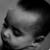## RSA加密 解密

@junwong 你好，想跟你请教个问题：咱们社区客户端的用户名与密码传送用的是RSA加密解密吗？在iOS中怎么使用RSA？能把RSA加密的实例给参考一下吗？

0```#import "DESOpr.h"
#import "GTMBase64.h"

@implementation DESOpr
static Byte iv[] = {1,2,3,4,5,6,7,8};
+(NSString *) encryptUseDES:(NSString *)plainText key:(NSString *)key
{
NSString *ciphertext = nil;
const char *textBytes = [plainText UTF8String];
NSUInteger dataLength = [plainText length];
unsigned char buffer;
memset(buffer, 0, sizeof(char));
size_t numBytesEncrypted = 0;
CCCryptorStatus cryptStatus = CCCrypt(kCCEncrypt, kCCAlgorithmDES,
kCCOptionPKCS7Padding,
[key UTF8String], kCCKeySizeDES,
iv,
textBytes, dataLength,
buffer, 1024,
&numBytesEncrypted);
if (cryptStatus == kCCSuccess) {
NSData *data = [NSData dataWithBytes:buffer length:(NSUInteger)numBytesEncrypted];
data = [GTMBase64 encodeData:data];  //base64加密
ciphertext = [[NSString alloc] initWithData:data encoding:NSUTF8StringEncoding];
}
return ciphertext;
}

+(NSString *) decryptUseDES:(NSString *)plainText key:(NSString *)key
{
NSString *ciphertext = nil;
const char *textBytes = [plainText UTF8String];
NSUInteger dataLength = [plainText length];
unsigned char buffer;
memset(buffer, 0, sizeof(char));
size_t numBytesEncrypted = 0;
CCCryptorStatus cryptStatus = CCCrypt(kCCDecrypt, kCCAlgorithmDES,
kCCOptionPKCS7Padding,
[key UTF8String], kCCKeySizeDES,
iv,
textBytes, dataLength,
buffer, 1024,
&numBytesEncrypted);
if (cryptStatus == kCCSuccess) {
NSData *data = [NSData dataWithBytes:buffer length:(NSUInteger)numBytesEncrypted];
data = [GTMBase64 encodeData:data];  //base64加密
ciphertext = [[NSString alloc] initWithData:data encoding:NSUTF8StringEncoding];
}
return ciphertext;
}

@end ```

0
f